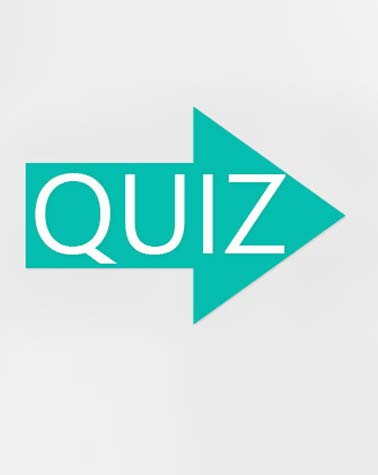# Integers Quiz!!

20 QuestionsSettingsTake this quiz to test out your knowledge of integers!

Related Topics
• 1.
Of the three integers below, which one is the smallest ?
• A.

-1000

• B.

3

• C.

0

• D.

-77

• E.

6

• 2.
What is the value of  ?
• A.
• B.
• C.
• D.
• E.
• 3.
What is the value of
• A.
• B.
• C.
• D.
• E.
• 4.
What is the value of  ?
• A.
• B.
• C.
• D.
• E.
• F.
• 5.
What is the value of  ?
• A.
• B.
• C.
• D.
• E.
• 6.
Which statement below  is   ?
• A.
• B.
• C.
• D.
• E.
• 7.
What is the value of  ?
• A.
• B.
• C.
• D.
• E.
• 8.
What is the value of ?
• A.
• B.
• C.
• D.
• E.
• 9.
What is the value of  ?
• A.
• B.
• C.
• D.
• E.
• 10.
What is the value of :
• A.
• B.
• C.
• D.
• E.
• 11.
What is the value of  ?
• A.
• B.
• C.
• D.
• E.
• 12.
What is the value of  ?
• A.
• B.
• C.
• D.
• E.
• 13.
What is the value of: ?
• A.
• B.
• C.
• D.
• E.
• 14.
What is the value of   ?
• A.

-15

• B.

15

• C.
• D.
• E.
• 15.
• A.
• B.
• C.
• D.
• E.
• 16.
In a game of darts, a player scores   points if he gets a bullseye,  points if he gets a red,  points if he gets a green, and  points if he gets a black.  If a player has 10 shots and scores:3 bullseyes, 1 red, 4 greens and 2 blacks, his final score would be:
• A.
• B.
• C.
• D.
• E.
• 17.
The height of Mt Everest above sea level is about 8850 metres. Danakl Depression in Ethiopia is   125 m below sea level. What is the difference in height between the top of Mt Everest and the bottom of the Danakl Depression?
• A.
• B.

• C.
• D.
• E.
• 18.
Which set of numbers is correctly arranged in ASCENDING order?
• A.

-157, -36, -2, 0, 1, 65

• B.

-1,21,333,-4655,-33267

• C.

-8, 80, -2, 567,1000

• D.

0, -2, -12, -45, -100

• E.

876, 456, 344, 27, -5

• 19.
Which number is equal to  ?
• A.
• B.
• C.
• D.
• E.
• 20.
In a skyscraper, one lift starts from the sixteenth floor,and travels up at the rate of  4 floors per minute. Another elevator starts on the tenth floor and travels down at the rate of 2 floors per minute.  How many floors are the two elevators apart after 5 minutes?
• A.

5

• B.

8

• C.

24

• D.

36

• E.

42